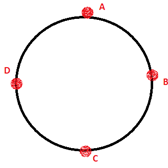Physics
Easy

Question

# The time taken for one complete circle is called the _____.

## Time periodFrequencyAngular velocityVelocityHint:

## The correct answer is: Time period

Physics

Physics

Physics

### A boy moves a tied stone above his head in circles, what is the time period of circular motion if it forms a circle of 20 cm radius. The speed of rotation is 23 m/s.Physics

Physics

Physics

### What is the direction of the velocity at point mentioned if the ball is revolving clockwise.At point CPhysics

Physics

Physics

### What is the velocity formula for uniform circular motion?Physics

Physics

Physics

### Which statement is true about an object that is moving in a uniform circular motion?Physics

Physics

Physics

### In a motion the magnitude of the velocity is constant, but the direction is not constant, and the force is acting towards the center. Identify the motion being talked about.#### With Turito Foundation.#### Get an Expert Advice From Turito.